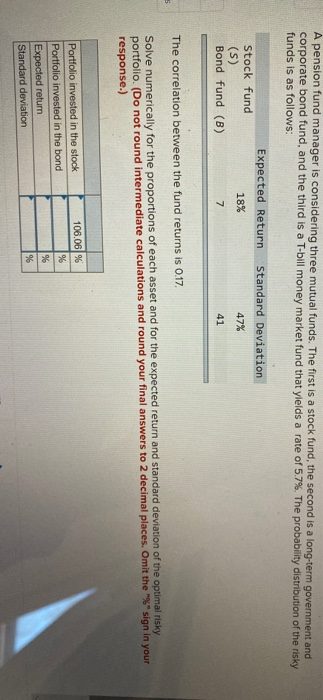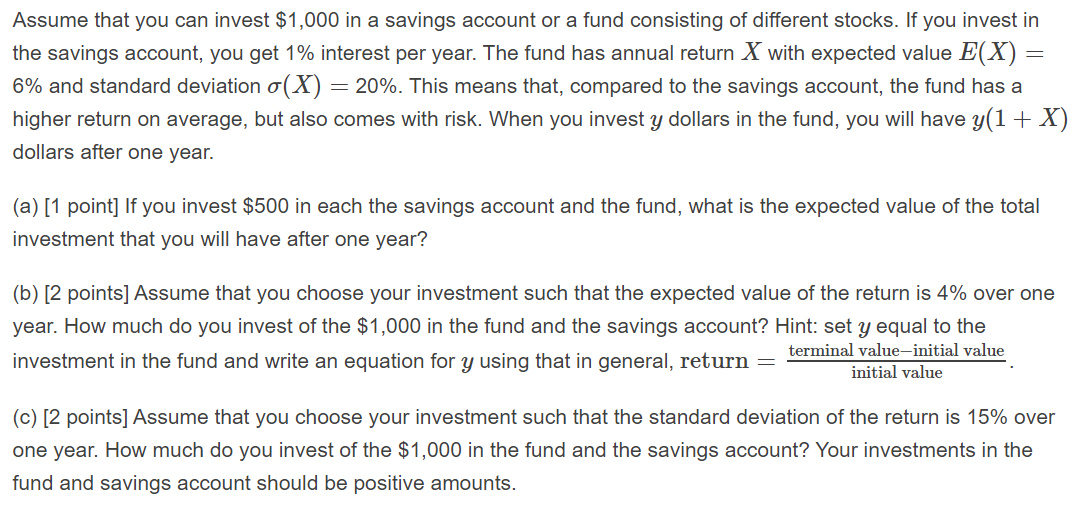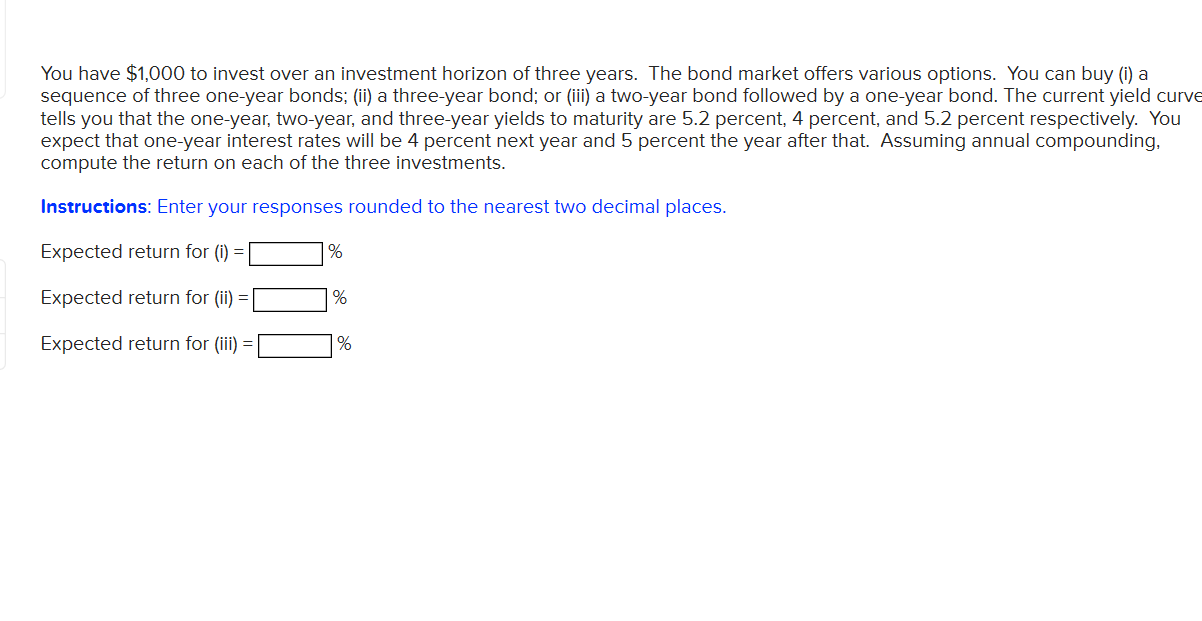# You have \$12,000 to invest, and three different funds from which to choose. The municipal bond...

You have \$12,000 to invest, and three different funds from which to choose. The municipal bond fund has a 7% return, the local bank's CDs have an 8% return, and the high-risk account has an expected (hoped-for) 12% return. To minimize risk, you decide not to invest any more than \$2,000 in the high-risk account. For tax reasons, you need to invest at least three times as much in the municipal bonds as in the bank CDs. Assuming the year-end yields are as expected, what are the optimal investment amounts?

Optimal amount in municipal bonds = \$

Optimal amount in CD's = \$

Optimal in high risk amount account = \$

Optimal return expected = \$

Let the amount invested in municipal bond be x

Let the amount invested in local CD's be y

Let the amount invested in high risk account be z

Maximize, Profit = 0.07 * x + 0.08 * y + 0.12 * z

Constraints:

x + y + z = 12000 [Total amount invested must be equal to \$12,000]

z <= 2000 [Maximum amount invested in high risk account should not exceed 2000]

x >= 3*y [The amount invested in municipal funds must be atleast three times greater than local CD's]

Solving this constraints, we get

z = 2000

x = 7500 and y = 2500

Optimal amount in municipal bonds = \$7,500

Optimal amount in CD's = \$2,500

Optimal in high risk amount account = \$2,000

Optimal return expected = 0.07 * \$7500 + 0.08 * \$2500 + 0.12 * \$2000 = \$965

Note - Post any doubts/queries in comments section.

Optimal amount in municipal bonds = \$6,000

Optimal amount in CD's = \$4,000

Optimal in high risk amount account = \$2,000

Optimal return expected = 0.07 * \$6000 + 0.08 * \$4000 + 0.12 * \$2000 = \$980

Note - Post any doubts/queries in comments section.

source: Exell
##### Add Answer of: You have \$12,000 to invest, and three different funds from which to choose. The municipal bond...
Similar Homework Help Questions
• ### You have \$55000 to invest, and two funds you would like to invest in

1. You have \$55000 to invest, and two funds you would like to invest in. The You-Risk-It Fund yields 13% interest. The Extra-Dull Fund yields 7% interest. Because of the college financial aid implications, you don’t think you can afford to earn more than \$6500 in interest income this year. How should you put in each fund?

• ### You have \$55000 to invest, and two funds you would like to invest in

1. You have \$55000 to invest, and two funds you would like to invest in. The You-Risk-It Fund yields 13% interest. The Extra-Dull Fund yields 7% interest. Because of the college financial aid implications, you don’t think you can afford to earn more than \$6500 in interest income this year. How should you put in each fund?

• ### You have \$55000 to invest, and two funds you would like to invest in

4. You have \$55000 to invest, and two funds you would like to invest in. The You-Risk-It Fund yields 13% interest. The Extra-Dull Fund yields 7% interest. Because of the college financial aid implications, you don’t think you can afford to earn more than \$6500 in interest income this year. How should you put in each fund?

• ### You would like to invest in \$12,000 in two different bonds

You would like to invest in \$12,000 in two different bonds. Some of the amount will be invested in treasury bonds at 10% annual interest and the rest in municipal bonds at 15%. What amount should be invested in each type of bond rate if the annual yield expected is \$1,440?

• ### A pension fund manager is considering three mutual funds. The first is a stock fund, the...

A pension fund manager is considering three mutual funds. The first is a stock fund, the second is a bond fund, and the third is a T-bill money market fund that yields a sure rate of 5.0%. The probability distributions of the risky funds are: (Total 20 points) Expected Return of Market Standard Deviation Stock funds (S) 15% 32% Bond funds (B) 9% 23% The correlation between the fund returns is 0.15. a. Use the formula below to compute the...

• ### You plan to invest \$12,000 in two funds paying 41/2% and 5% simple interest

You plan to invest \$12,000 in two funds paying 41/2% and 5% simple interest. (There is more risk in the 5% fund.) Your goal is to obtain a total annual interest income of \$580 from the investment. What is the smallest amount you can invest in the 5% fund and still meet your objective?

• ### Need a help please. Thank you. A pension fund manager is considering three mutual funds. The...Need a help please. Thank you. A pension fund manager is considering three mutual funds. The first is a stock fund, the second is a long-term government and corporate bond fund, and the third is a T-bill money market fund that yields a rate of 57%. The probability distribution of the risky funds is as follows: Expected Return Standard Deviation 47% 18% Stock fund (S) Bond fund (B) The correlation between the fund returns is 0.17. Solve numerically for the...

• ### 3. You have a risky portfolio that yields an expected rate of return of 15% with...

3. You have a risky portfolio that yields an expected rate of return of 15% with a standard deviation of 25%. Draw the CAL for an expected return/standard deviation diagram if the risk free rate is 5%. a. What is the slope of the CAL? b. If your coefficient of risk aversion is 5, how much should you invest in the risky portfolio? 4. A pension fund manager is considering three mutual funds. The first is a stock fund, the...

• ### Assume that you can invest \$1,000 in a savings account or a fund consisting of different...Assume that you can invest \$1,000 in a savings account or a fund consisting of different stocks. If you invest in the savings account, you get 1% interest per year. The fund has annual return X with expected value E(X) = 6% and standard deviation o(X) = 20%. This means that, compared to the savings account, the fund has a higher return on average, but also comes with risk. When you invest y dollars in the fund, you will have...

• ### You have \$1,000 to invest over an investment horizon of three years. The bond market offers...You have \$1,000 to invest over an investment horizon of three years. The bond market offers various options. You can buy (i) a sequence of three one-year bonds; (ii) a three-year bond; or (iii) a two-year bond followed by a one-year bond. The current yield curve tells you that the one-year, two-year, and three-year yields to maturity are 5.2 percent, 4 percent, and 5.2 percent respectively. You expect that one-year interest rates will be 4 percent next year and 5...

Free Homework App